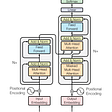# Monopsony Theory

In my first blogpost (here) I connected some equations related to the economics of monopolies and applied them to labor markets. I ended up focusing on a value which I called the (labor) price elasticity of demand for money, and thought that it was a representative measure of Marx’s surplus value (1). If this value was inelastic, then the firm would be able to pay workers less without losing any workers. Thus a firm would be able to profit more and pay workers less if the workers lived in conditions of precarity.

As it turns out, this kind of analysis is quite old. In retrospect, it is not surprising that you could take monopoly theory and just apply it to the labor market. This analysis seems to generally fall under neoclassical economic theory, typically under “monopsony theory”. I was unaware of the naming convention at the time; the elasticity term I focused on is known as the “(wage) elasticity of labor supply”. Otherwise, it basically lines up 1:1 with the math from that blog post.

However, there is a strange way economists go about writing about monopsony/monopoly. In fact, when I first encountered monopsony theory I did not realize that I had already done the exact same math. I could tell the monopsony math was technically correct, but the language used in the explanation did not seem to line up with the math. In fact, the economists seemed to come up with the opposite conclusion as I did. Milton Friedman called monopsony a “theoretical curiosum” (2): it had no bearing on the real economy. Whereas I took it to represent the Marxian concept of surplus value, effectively underlying all of labor in the economy.

So how did we end up with two completely different views on the exact same mathematics?

As it turns out, Joan Robinson (who created the monopoly/monopsony framework used today) explicitly referred to to it as the analysis of exploitation; a section of her book is titled “Book IX Exploitation” (3). So what happened since then, such that modern economics texts treat monopsony the same way Friedman does instead of similar to Robinson?

## Some Math for Context

It seems all educational texts on monopsony follow the same general outline. I will be referring to these (2,4,5,6). For notation, I will use this text (4) from Moscow University, which has the mathematical derivations for the graphs that’ll be used throughout the post.

Above is a firm’s profit equation. Here, the firm is trying to maximize its profit (PR). What it can do is vary the amount of labor (L) that it purchases. Fixed non-human capital (r*K and FC) are constant with respect to labor L. Every other term is a function of L (I will try to emphasize this by adding (L) where it’s not confusing). The wage per unit labor is w (or w(L)), so the total amount of money paid out to laborers is w*L (w(L)*L). Revenue is the price of the product p(L) multiplied by the quantity of product sold Q(L).

Take the derivative of profit equation with respect to L (equate to 0 to obtain optimal/maximal value).

Terms involving p and Q encompass the product market and will generate a downward sloping curve (each new unit of labor acquired will increase the amount of product the firm can make, but demand for the product becomes less and less, as is usual for a demand curve). These terms (pdQ/dL + dp/dLQ)can be rewritten as MR*MP(L) (also known as MRP(L), the marginal revenue product of labor).

So the derivative will generally be rewritten like this:

The terms w+(dw/dL)*L correspond to the labor market. These generate another curve which corresponds to the marginal cost to the firm. From these terms we can imagine two separate curves. Firstly, a curve just involving w(L), this is known as the supply S. We can also write a curve involving both terms w+(dw/dL)*L, this is known as the marginal factor cost curve MFC.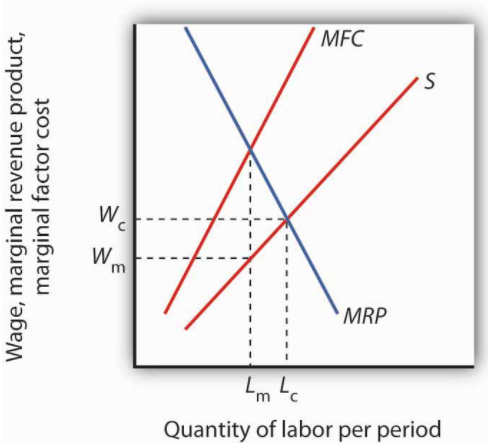Figure 1. Marginal factor cost, Supply and marginal revenue of product (from 5)

Where the MFC and MRP curves intersect (MR*MP(L)=w+(dw/dL)*L), this will satisfy the conditions for maximal value (the derivative of the profit equation equals 0). In the figure above, this optimal value is at Lₘ. The wage paid out per unit labor at the optimal value is thus w(Lₘ), which is Wₘ.

(Slight manipulation here gets the wage elasticity term, as discussed in my first blog post. It’s not too important here since dw/dL is the more interpretable term, just know that when dw/dL is 0, then the wage elasticity is perfectly elastic; i.e.(negative) infinity.)

If the wage is constant with respect to L, then dw/dL equals 0 (that is, w(L) is a constant horizontal line), and thus (dw/dL)*L equals 0. This reduces our optimal equation to just:

Famously, this states that the wage of labor will equal the marginal revenue product of labor. Generally considered one of the backbones of free market fundamentalism.

When does dw/dL equal 0? Well this happens under perfect competition in the labor market, which leads to my first main criticism with the mainstream portrayal of monopsony.

## 1. The Perfect Competition Assumption

There seems to be a strange habit in econ to assume that the real world is most similar to the perfect competition model over other models. Joan Robinson commented about this at the time (8):

… unless competition is perfect the factors do not receive the value of their marginal product as wages. It was to avoid this difficulty that he took refuge in the assumption of perfect competition

In every single one of the modern educational texts on monopsony (2,4,5,6,7) seems to use the perfect competition assumption. That is, it is fair to assume that w(L) is constant, thus dw/dL=0 and the elasticity of labor supply is infinite (perfectly elastic).

Of course the alternative is non-perfect competition; w(L) is not constant, dw/dL is not 0, and the elasticity of labor supply is not infinite. This is called imperfect competition and is in fact the title of Joan Robinson’s book (“The Economics of Imperfect Competition”). Robinson was actually the first to introduce the mathematics for a market where dw/dL is not 0. Though her methods we’re relied on geometry, it was basically equivalent to the math described above. Robinson explains that the real world markets are not in perfect competition, so she uses monopoly/monopsony analysis to describe them. For her, all real world markets have some degree of monopoly/monopsony, and perfect competition is a theoretical limit that never occurs in real life. In her analysis, monopoly/monopsony is imperfect competition.

This is where the terminology gets confusing because a “true monopsony” refers to a market involving only one supplier. So it’s reasonable to assume that Robinson’s monopsony analysis only refers to the case where there is only one supplier. But, this is incorrect; as the math in the previous section shows, Robinson’s analysis describes everything outside of perfect competition. For some reason or another, modern teaching texts have used this confusion to pretend that the monopsony analysis /”monopsony power”(dw/dL not 0) is only valid when there is a “true monopsony”.

In fact, all the texts I checked out end up using the term “competitive market” as shorthand for “perfectly competitive market” (2,4,5,6,7) and in contrast to Robinson’s “imperfectly competitive market”. They go to great lengths to imply that perfect competition is a reasonable reflection of the real world, and that dw/dL is generally 0 because there are so few “true monopsonies” in the real world. This is backwards; dw/dL (and labor elasticity) can give you a sense about how competitive the labor market is, but the amount of competition in the labor market does not dictate the value of dw/dL. You can easily have an inelastic labor market even if there are many employers in the space (10,11,12).

Mankiw’s Principles of Economics (I believe the most used econ textbook,7) literally avoids monopsony, despite having an analysis of monopoly. It does not present anything to similar (Figure 1), no mention of MFC, dw/dL, etc despite presenting the equivalents in monopolies. It states this:

This book does not present the formal model of monopsony because, in the world, monopsonies are rare. In most labor markets, workers have many possible employers, and firms compete with one another to attract workers. In this case, the model of supply and demand is the best one to use. (7)

Compare this to Joan Robinson’s analysis that showed that

the exploitation of labour is endemic within modern economies as a result of the prevalence of monopolistic and monopsonistic elements (9)

(I should note that some of the texts are more fair to monopsony analysis than others, most weren’t as bad as the Mankiw book, but none imply its endemicity like Robinson did.)

I want to stress, perfect competition requires that dw/dL is 0 (perfectly elastic). It is strange to assume perfect elasticity, it would make more sense to assume some degree of inelasticity, and then figure out what that degree is.

In fact there’s been tons of empirical evidence (10,11) has shown that dw/dL is far from 0 and that the labor market is inelastic (a far cry from perfect competition) even when there are plenty of suppliers of labor. There is no reason for the perfect competition assumption.

## 2. The Supply Curve

Introductory Econ will teach students that the supply curve for a product is generally upward sloping. Of course everything is more complicated, but it’s good intuition. Upward sloping supply curves tells you the limits on resources for a person/firm/country. As you purchase more of a resource and the resource becomes more and more scarce, it will be harder to obtain some of the remaining resource.

It would give you quite a bit of whiplash to find a certain situation requires that the supply curve to be flat (non-upward sloping), right? Of course, that’s exactly the situation described by perfect competition. dw/dL = 0 means that w(L) and MFC(L) are horizontal; this means non-upward sloping supply curve.

I can’t prove to what extent the economics field deliberately goes to obfuscating this contradiction, but I can give some examples of how odd it makes the texts.

Figure 2 from the Moscow University text. On the right you see horizontal supply curve, and the equilibrium point where it crosses the demand curve. It’s strange isn’t it? Not your typical supply and demand. So how is it rationalized?

That’s done by the figure on the left. What happens in perfect competition is the market is actually occurring in the far off distance, and the individual firm is unable to influence it no matter what. It’s this great far away market that sets the wage/price, and then the individual firm is only able to pay for labor at this price. It’s termed a “price taker”.

This is such a hilarious sleight of hand, I won’t be surprised if they don’t use it elsewhere. What they’ve essentially done is justify an unfavorable account of supply and demand by pretending there’s actually supply and demand going on somewhere else, and that’s fixing the wage price. It’s obviously much more intuitive to just have an upward sloping supply curve.

This leads to some genuinely incorrect mathematical statements. For example, most of the teaching texts will show a figure similar to Figure 1, and then ask the reader “What would happen if the market was (perfectly) competitive?” The answer that’s given is that the MFC curve would disappear and the profit maximizing point would be where the S curve crosses the MRP curve. (5,6, see 13 for an example)

This is just mathematically incorrect, increased competitivity means lowered S curve slope, and perfect competition means the supply curve must be horizontal, this part of the usual definition of perfect competition (see Figure 2). You cannot increase competition and keep the S curve the same, and you cannot change/remove the MFC curve without changing the S curve.

For example, take the S curve as a straight line with slope a and intercept b.

S(L) = w(L) = a*L + b

MFC(L) = w(L) + (dw/dL)*L
……….= a*L + b + (dw/dL)*L
……….= a*L + b + a*L
MFC(L) =2 a*L + b

So the MFC curve will always have twice the slope of the S curve (if it’s a straight line). The only time it “disappears” is in perfect competition, where it lies exactly on top of the now horizontal S curve. There’s also no guarantee that the horizontalized S curve under perfect competition would cross the MRP curve at the same spot as it did before.

I’m not sure to what extent this is some deceptive way to that perfect competition and upward sloping supply curves are compatible, or just a genuine mistake that’s repeated. At the very least it is an example of the difficulty of working through these two contradictory pillars of economics.

I should note that this is all consistent with the definition of perfect competition; which states that the individual firm is so small in the grand scheme that it has no bearing on price/wage. But, this should be a huge clue to whether the perfect competition assumption is useful. If you are taking perfect competition to be true, you are saying the supply curve is flat.

Originally another section here, but this post has gotten too long. I’ll just lay out some other thoughts in point form:

1. The perfect competition wage=MPR leads to an false intuition that there is a direct causal connection between a worker’s wage and the revenue they create for the firm. It would be better to think of the wage function and revenue completely separately, connected through the firm but only as minor factor. The main driver of wage, in my view, is the precarity of the worker and what they are willing to accept.
2. Economists seem to think that dw/dL and the elasticity of labor supply are purely a function of the degree of market competition. There is a connection there, but many things have influence here, including worker precarity/bargaining power.

The perfect competition assumption has led to pretty bad takes about the world. Most notably it has been used to demonstrate the non-viability of a minimum wage. Milton Friedman’s “theoretical curiosum”(2) comment was in reference to the idea that setting a minimum wage could actually increase employment. I haven’t shown it here, but this is a prediction that occurs in monopsony analysis, but does not occur in perfect competition. And Friedman was not alone, most economists agreed with him at the time.

Of course, it’s been shown that a minimum wage can increase employment (10), and yet economists rejected that finding; preferring their theoretical perfect competition over what they considered to be statistical noise.

And in a larger set of studies, it’s been shown that the minimum wage increased worker pay while not changing the level of employment at all. Similarly, studies have shown that many labor markets are highly inelastic, thus highly nonperfect and highly exploitative (12).

This extends beyond the minimum wage too; many labor market initiatives are opposed by economists because they view the labor market as Friedman did. For example, as the covid pandemic recedes, many are already arguing for the cutting of unemployment insurance on the grounds that UI constitutes a give away to those unwilling to work. This view arises from the perfect competition assumption, where the distribution of wealth is closely connected to the value of labor.

Lastly I should note that I’m not too particularly anchored to Joan Robinson’s monopsony analysis either. These are all just models after all. They are simplifications and starting points. But we should recognize that these models have a huge effect on your perception of the world. It is no surprise to me that the perfect competition assumption is so prevalent while the economics field is one of the most right leaning fields in academia. Imagine how different the world would look to students if, instead of the harmony of perfect competition, they are taught to view the world as exploitation.

Footnotes:

1. It turns out that the reciprocal of the elasticity of labor supply has been called the rate of exploitation/rate of surplus value (see the Welfare implications section of the Monopsony wiki page). Though this is the only page I’ve found that has argued this.
2. Milton Friedman, Price Theory, p 193
3. Joan Robinson, The Economics of Imperfect Competition, p 280.
4. https://www.econ.msu.ru/ext/lib/Category/x48/x05/18437/file/unit%209.pdf
7. Greg Mankiw, Principles of Microeconomics, 5th edition page 406
8. Joan Robinson. (1933b) ‘The Co-ordination of the Laws of Distribution: A Review’, Economic Journal, 43: 301–304.
9. Paul Flatau, ‘Some Reflections on the ‘Pigou-Robinson’ Theory of Exploitation’, page 7.
10. David Card and Alan B. Krueger. Famous for their 1995 study: Minimum Wages and Employment: A Case Study of the Fast-Food Industry in New Jersey and Pennsylvania. And for their book: Myth and Measurement: The New Economics of the Minimum Wage.
11. https://www.princeton.edu/~tleonard/papers/minimum_wage.pdf
12. See Arindrajit Dube’s work: https://arindube.com/published-articles-and-book-chapters/
13. Example incorrect statement (below the figure) about the MFC(MRC)/MRP curves. Taken from 6. As stated in the blog, the S curve cannot remain the same under perfect competition, it must be horizontal. And given that it’s horizontal, there’s no guarantee the horizontal S curve intersects MRP at the same point as the monopsonistic S curve. In fact, to assume that it does breaks the prior assumption that the individual firm has no affect on the wage under perfect competition.

--

--

--

## More from Phil N

Love podcasts or audiobooks? Learn on the go with our new app.

## Neural Quine: Is Self-Replicating AI Real?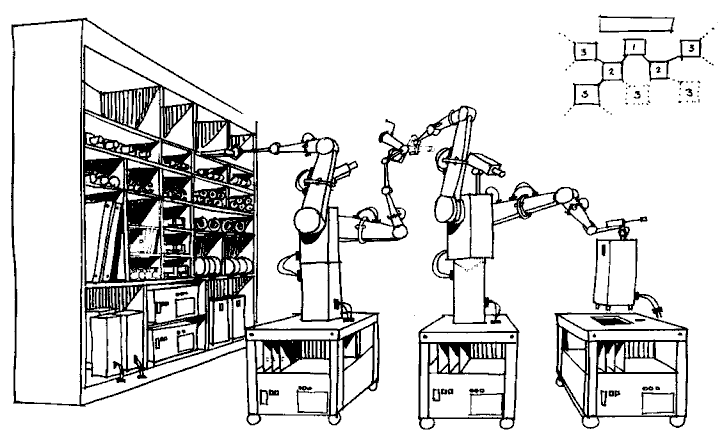## The Results of the WETH/USDC Limit Farm’s Second Round## #23 Can You Solve This Simple Math Problem?## Basics of Linear Algebra for machine learning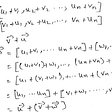## Why a⁰ = 1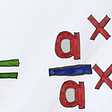## How To Design A Franc-Carreau or Fair-square Game## What is Error Correction Coding? Hamming? BCH?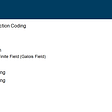## Why Does Temperature Have No Upper Limit?## Ethical AI## Incongruity and Hijab## Applying Robotics in Pandemics